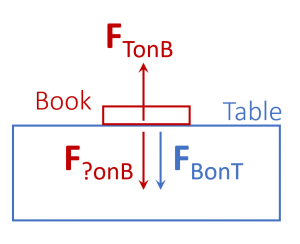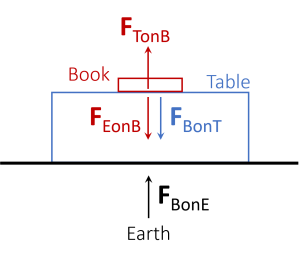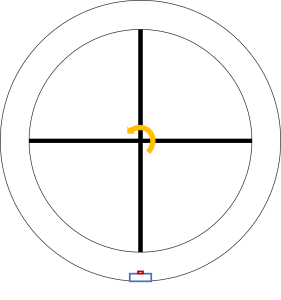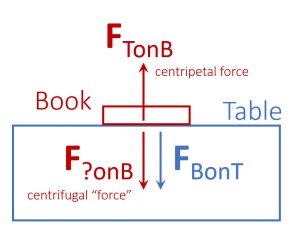# Is the centrifugal force real?

Yes and no.

My shortest post yet!… or not?

I should probably write a bit more – maybe revisit part of a presentation I gave at a physics teachers’ conference. Then again, when I was a school student a physics teacher insisted “The centrifugal force is not real!” Yet he did little more to help me understand what he meant.

However, rather than whining about the past, I should probably help teacher’s who might similarly lack useful tools in this situation, or who want to add further to their toolbox.

OK… so I’ll provide a relatively simple instructional tool that I’ve not seen used elsewhere – it links to a commonly used example, and other important instructional tools.

Yes… the individual ideas are far from original, and the approach may even have been presented exactly like this elsewhere. However, if it has, I’m not aware of it, and it bears repeating.

Note: this is aimed at teachers (to whom I apologise if I’m sometimes too pedantic in spelling things out) but others are, of course, free to learn from it. A teacher would need to “package it” to use as a “lesson” i.e. remove my digressions and comments, appropriately guide students through the scenarios, get them to draw the diagrams (in a manner they’re familiar with), add the forces etc.

## Motivation

I was prompted to write this by a recent twitter poll (as well as some related posts by @MrJoeMilliano):

Given my answer at the beginning of this post, I wasn’t surprised by the poll’s results.

To be clear… I know I’m not the first to point out that “Yes” and “No” are both valid answers. Or, equivalently, that the question (along with either a “Yes”, or “No”, answer) by itself, is not particularly helpful in understanding the related physics.

However, the following exploration (which involves two, almost identical, scenarios) might help clarify / help you clarify for others, why the question is ambiguous.

## Scenario 1 – relatively simple

Imagine your in a room with no way to see, or communicate with, the outside world – I’m riffing on Galileo’s ship (as many have) i.e. the bit about being shut up in a cabin.

In the room there’s a book on a table, as shown in the diagram below – a classic situation found in “every” physics textbook / presented in “every” physics class.Figure 1 – The forces related to a book sitting on a table in the room.

So let’s discuss the forces in Figure 1.

If we “drop” the book, it accelerates towards the floor until it lands on the floor. So, there’s a force on the book due to something (we don’t know what yet) that if the table wasn’t there would cause it to accelerate towards the ground. I’ve labelled this force F?onB. The question mark indicates we don’t yet know what’s causing the force on B (the book).

[I’ve mentioned the utility of notation like FAonB in the post A force is not just a vector. The use of the notation here, is just one example of it’s utility.]

NOTES

1. The table exerts a force on the book FTonB. which is equal in magnitude and opposite in direction to F?onB. We can express this as FTonB = –F?onB. So, as any physics teacher knows, the book is not accelerating because all the forces “onB” balance / have a vector sum of zero / net force is zero (NOT because FBonT = –FTonB, which is always true by Newton’s 3rd law):

2. FTonB is often referred to as the “normal force”. Sometimes confusingly referred to as the “normal reaction force”… “confusingly” because it’s not the (Newton’s 3rd law) “reaction” force to F?onB, which is a misconception students sometimes hold.

3. The fact we can express the multitude of forces “by the table on the book” as a single force (the normal force, FTonB), acting at a point, is usually not explained/explained well… I might expand on this in another post.

4. To determine the (Newton’s 3rd law) “reaction” force to FTonB, we simply swap the objects in the subscript. i.e. it’s FBonT, which is shown in the diagram. So using this notation it’s “obvious” (and/or it’s simple for a teacher to point out) that FTonB is not (Newton’s 3rd law) “reaction” force to F?onB.

5. To avoid confusion around “reaction” (including students thinking that one of the “3rd law force pair” is specifically the “action” and the other the “reaction”) you can always word Newton’s 3rd law without mentioning “action/reaction” e.g. something like, in short form:

All forces exist as pairs.

In long form something like:

If there exists a force FAonB between two objects A and B, then there exists a force FBonA that’s equal in magnitude and opposite in direction to FAonB. We can express this as: FBonA = –FAonB.

However, student’s are possibly going to come across a resource with “action/reaction” mentioned. So one likely needs to tackle this at some point.space to be deleted

Now we open some shutters that were blocking a window, and we see… rolling hills outside. We’re on Earth! So we can update our diagram:Figure 2 – The forces related to a book sitting on a table in the room. We now know the room is on Earth.

We identify the force F?onB as the gravitational force due to the Earth. So we rewrite it as FEonB. We’ll also add in the (Newton’s 3rd law) force FBonE. As already mentioned, this is really easy to do using this notation because we just reversed the letters in the subscript – the Earth exerts a gravitational pull (attraction) on the book, and the book similarly exerts a gravitational pull (equal in magnitude, but opposite in direction) on the Earth, so FBonE = –FEonB.

So far so relatively simple. Let’s repeat, but with a twist.

## Scenario 2 = Scenario 1 with a twist

So, imagine your in a room with no way to see / communicate with the outside world, and there’s a book sitting on a table – you should be getting some serious deja-vu!

We have the same diagram that we started with previously, with the same forces.

However, this time you open the window shutters and look out to see that… you’re in space!… far enough away from any gravitating bodies that F?onB is clearly not gravity.

On further investigation, it turns out you’re in a rotating space station (Figure 4) – you can use your own imagination as to how you got there. For example, I think alien abduction to help you learn physics is a more appealing story (and a more believable one) than, say, alien abduction for probing.Figure 4 – Table with book in a ring spacestation rotating in the direction of the yellow arrow – clearly illustrating my lack of artistic talent!

Anywho, you realise (Figure 5) that the force F?onB is the centrifugal “force”. We can also identify FTonB as the centripetal force, a force directed towards the centre of rotation.Figure 5 – Identifying the centripetal and centrifugal forces.

FBonT is (Newton’s 3rd law) “reaction” force to this centripetal force. This force is sometimes incorrectly identified as the centrifugal “force”.

Possible question for students: “What speed of rotation would you need to simulate Earth’s gravity?”… yes, I know, we haven’t specified the size of the space station. It’s often good not to spell everything out. Get them to realise this, figure out whether it matters to the answer, decide what factors might determine a desirable/reasonable size etc.

## A pseudo-force

In the rotating frame of reference F?onB is clearly a “force” in the sense that it’s a “pull” that you and other objects feel towards the floor of the room. It’s analogous to the pull of gravity that we felt when we were in the room on Earth – the whole point of the design of the space station is to simulate gravity.

This is why I would suggest it’s far less confusing to refer to the centrifugal “force”as a pseudo-force (a force, of sorts) rather than just claiming “it’s not a force”.

Of course, we then need to be clear exactly why we’re using the prefix “pseudo”.

Note: other words such as “fictitious” and “inertial” are sometimes used instead of pseudo. While I don’t mind the term “fictitious”, I’m familiar with using “pseudo”, and “fictitious” is longer, and I’m lazy efficient. However, I dislike the term “inertial” because it can lead to students talking about “inertia” as a force. If there are common alternatives we know can avoid such misconceptions, then it would be obstruse not to use them!

## So why is the centrifugal force a “pseudo-force”?

If we make it clear that a force requires the following for it’s definition (see A force is not just a vector):

• The object on which the force is acting.
• The object causing the force.
• The magnitude of the force…
• …which, for quantitative work, requires us to choose a unit (commonly newtons).
• The direction of the force (essentially, is it a push or a pull?).

then it’s relatively easy to explain that:

For a “centrifugal force” there’s no object causing the force i.e. in Scenario 1 we could replace the “?” with “E”. In Scenario 2 there’s nothing to replace it with.

So, the centrifugal “force” is not a “force” in the full sense of a force. It only partially meets our understanding of what a force is, hence “pseudo-force”.

In contrast, the centripetal force can be “fully” specified e.g. when the book is sitting on the table we have FTonB (both objects) and so we also have the “reaction” force FBonT.

## Additional key points

A result of this, is that:

• The centrifugal pseudo-force has no “reaction force” i.e. in Scenario 2 we cannot swap the ? and B around. There is no object for the book to exert a (reaction) force on.
• The centrifugal pseudo-force “disappears” in an inertial reference frame. From the perspective of an inertial reference frame, watching the spinning space station from outside, there is no such force (in contrast, FTonB and FBonT still exist).

(It could be said that these are the defining features of a pseudo-force… I’ll discuss Newton’s laws and the difficulty of rigorously/coherently defining them, and forces etc. in another post.)

The second dot point relates to the different stories (in the different reference frames) around: the book “falling” to the ground when it’s “dropped”.

• Within the rotating frame the force appears to be similar to gravity.
• In an inertial frame, looking at the space station from outside, the story is different. When a centripetal force is no longer applied to the book by the table (or our hand etc.) the book moves in a straight line (Newton’s first law).

In this frame what appears to be the book falling “straight down” (to someone in the space station) results from the floor of the space station rotating to meet it. When the book is on the floor, the floor then provides the centripetal force that, once again, means the book is rotating in a circle with the rest of the room – feel free to draw diagrams illustrating this that are much better than any I would draw!

## Remembering the difference

It’s relatively easy to remember that the centr-ipetal force is a force towards the “centre” of the rotational motion. Also, it’s a centRipEtAL (“real”) force – OK, someone can probably come up with something better than that!

Centrifugal, is the opposite. In the rotating reference frame it points away from the centre and it’s a pseudo-force (“not real”).

## So is the centrifugal force real?

If by “real” you mean “Is it a force that someone can experience?” (a push or a pull) then, yes. It is.

If by “real” you mean “can we technically specify the force as described in A force is not just a vector“, or similar, then the answer is no.

Is there an existing, common way to describe a centrifugal “force” to help avoid this ambiguity?… yes. We can refer to the centrifugal “force” as a pseudo-force (or fictitious-force).

I guess one could also call it the “centrifugal effect” as people sometimes refer to the “Coriolis effect”. I would suggest that you are at least consistent (e.g. use “centrifugal pseudo-force” and “Coriolis pseudo-force”, or “centrifugal effect” and “Coriolis effect”) but also mention the alternatives students will likely come across.

### Can we agree on a single term?

I’m voting for pseudo-force (over “fictitious-force” or “effect”). Maybe I’ll run a twitter poll and post the results here?!

However, I’ve possibly already “lost” because the related Wikipedia page is titled “fictitious force”… on the other hand I haven’t done an extensive check on all web sites, or an exhaustive survey of textbooks, to know what the most widely used term is. Also, I’m with Feynman on this one (he uses pseudo-force)… a powerful ally!

Most frustratingly, Wikipedia refers to “inertial force” in several places… sure, maybe mention it as a historical term… but also point out it can be misleading.

## Interesting extensions

### Differences

One interesting extension is to discuss how the two situations differ, e.g. if we extended the size of the room / area under consideration i.e. what’s different about the forces as we go “up” in both scenarios?… what’s different if we expand our horizons sideways?… etc.

This does indicate that, depending on the sensitivity of our measuring instruments / the size of the room, we might have been able to tell the difference between the two scenarios before opening the shutters. Of course, in our scenarios we’re assuming the area we’re considering is small enough that there are no differences to the precision that we can measure.

This leads to another interesting extension…

### Gravity

Our scenarios effectively relate to Einstein’s equivalence between acceleration and gravity (over a small enough area). Although, commonly, one compares the simpler linear acceleration of “an elevator” to gravity.

How can we easily transition from here to general relativity in a more meaningful way than just saying that “gravity is not a force?”. For example, it would appear to be “not a force” in a different sense to the centrifugal force not being a force. However, that’s for another post (otherwise this one will just keep going and going!).

## Other centrifugal examples

The importance of this example, is as an initial example, and a reference for other examples. Following this example, one should get students to do a similar analysis of other examples – turning the corner in a car, riding a roller-coaster around a loop-the-loop, the motion of objects on a spinning disk etc.

### Why is this example particularly useful?

This example is particularly useful as an introduction because of the explicit comparison between the “real” gravitational force and the centrifugal force.

In the scenario, for example, of a car turning the corner, there’s no equivalent force with which to compare and contrast the centrifugal pseudo-force. I would suggest this sometimes leads to incorrectly identifying, for example, the (Newton’s 3rd law) “reaction” force of the centripetal force, as the centrifugal force.

Having the space station example to refer to is useful in understanding these other examples. It definitely helps me!

Also, rotating space stations generating artificial gravity are a common sci-fi trope e.g. anyone played Halo? And so they make an excellent, “familiar” (for some) example to work with. For those not into sci-fi, it’s also a nice real-world scenario. Such artificial gravity has been considered for real space-stations / space-ships etc. In general, rotational dynamics in space is really important (and this is a relatively simple introduction to it)… and sometimes particularly intriguing e.g. in the must-see Veritasium video The Bizarre Behaviour of Rotating Bodies.